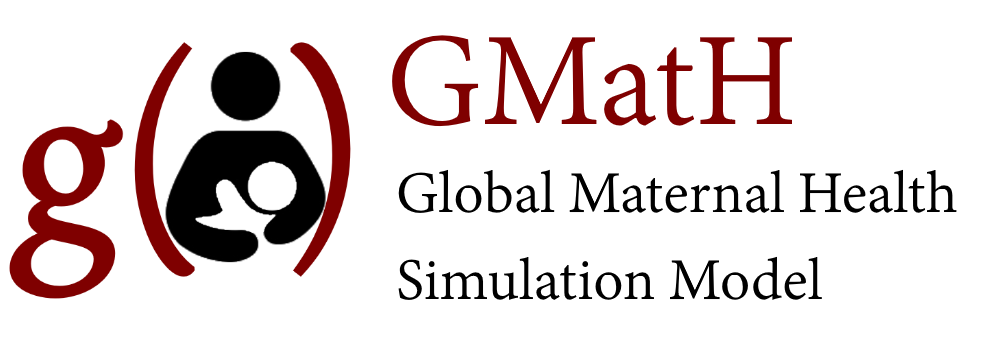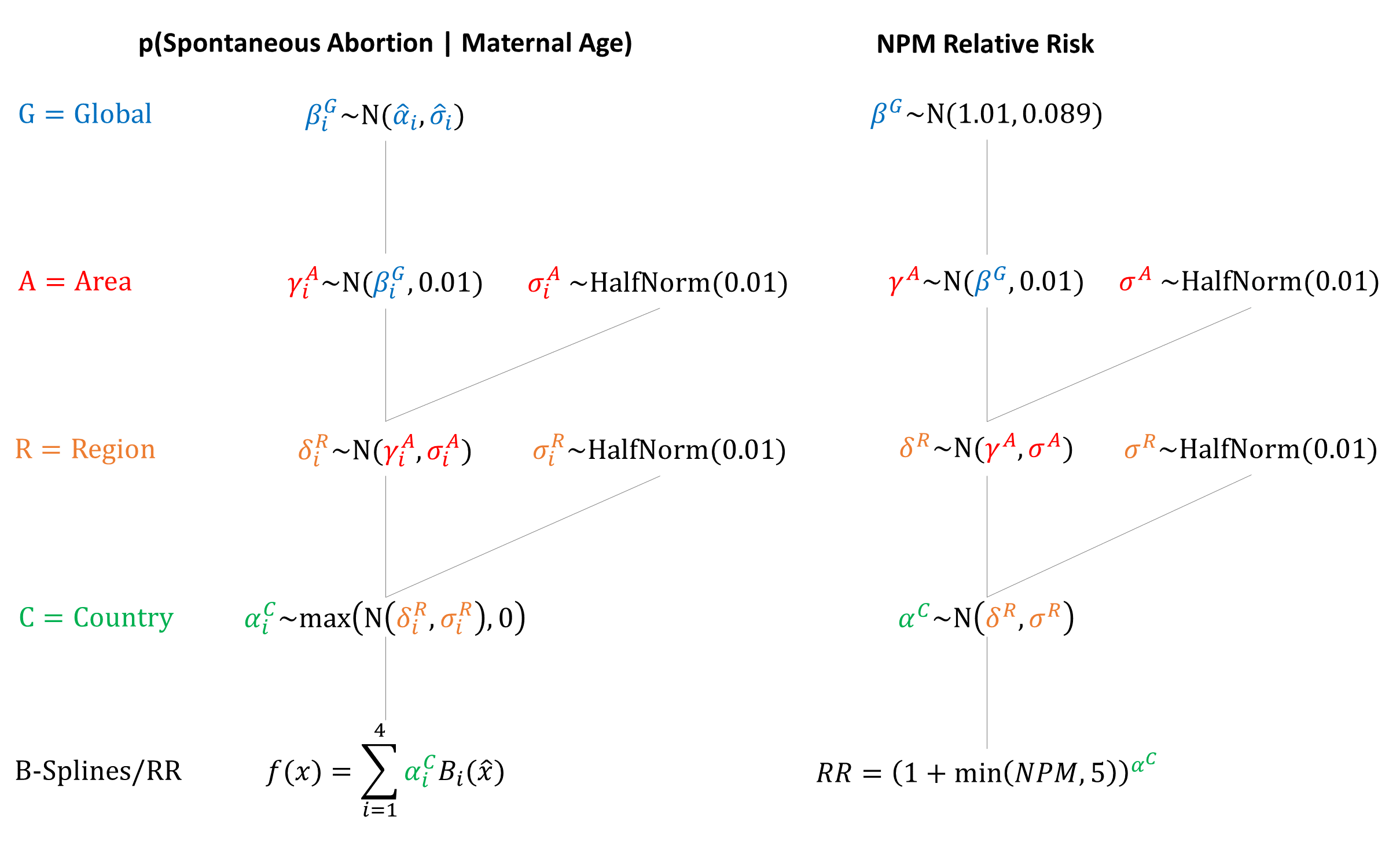Model Inputs $$\rightarrow$$ Biological Parameters $$\rightarrow$$ Miscarriage

### Overview

The term ‘miscarriage’ in the model refers to spontaneous abortion. Ectopic pregnancy is modeled separately as a high risk condition with risks of morbidity/mortality. In contrast, we assume that spontaneous abortion poses no physical risks to the mother.

### Data

Maternal age is a strong predictor of miscarriage. Andersen (2000) presents rates of spontaneous abortion by maternal age group from a population-based register linkage study in Denmark. After adjusting the denominator by subtracting all induced abortions from the number of pregnancies, the adjusted risk of miscarriage was calculated by age group. These estimates are thus conditional on no induced abortion.

The risk of miscarriage given previous miscarriages is also modeled, to take into account within-women clustering/high-risk women. Estimates were modeled using data from Risch 1988, which estimated a Relative Risk of miscarriage based on the number of previous miscarriages ($$NPM$$). The equation is: $$(1+NPM)^{\alpha}$$, where $$\alpha$$ was estimated to be 1.01 (95% CI 0.83-1.18). Some of the estimated increased risk is likely due to older age, so we use calibration to estimate the overall risk of miscarriage, taking into account maternal age and previous number of miscarriages.

A systematic review by Avalos et al. (2012) reported risk of miscarriage by gestational age up to 20 weeks gestation. The distribution of gestational age at miscarriage across the 4 sources[4,5,6,7] was averaged in order to estimate the distribution of gestational ages at which miscarriages occur. All miscarriages are assumed to occur by 6 months gestation. The midpoint (in weeks) of month of gestation was used and the following distribution is assumed in the model:

Month of Gestation Cumulative Probability of Miscarriage | Miscarriage
2 0.2262
3 0.5866
4 0.8824
5 0.9684
6 1.0

Estimates of miscarriage in early pregnancy are especially difficult to ascertain as women may not know that they are pregnant. We therefore begin modeling the risk of miscarriage in the second month of gestation.

### Parameters

We fit quadratic B-splines to the midpoints of the age groups. Spline Width: 40

Spline # Knot Location Height
1 8 $$N(0.15,0.02)$$
2 22 $$^1/_3$$ $$N(0.09,0.02)$$
3 37 $$^1/_3$$ $$N(0.14,0.02)$$
4 52 $$N(1.15,0.08)$$

We used the estimated probability of miscarriage at age 50 for older ages.

To model increased risk given a history of miscarriage, we base our prior on Risch 1988, which estimated $$\alpha$$ to be 1.01 (95% CI 0.83-1.18), which translates to $$\alpha \sim N(1.01, 0.089)$$. A maximum of 5 previous miscarriages is used when estimating increased risks of miscarriage.### Model Implementation

Following conception in the model, the risk of miscarriage is simulated based on a woman’s age and her history of previous miscarriages. If a miscarriage is simulated to happen, the gestational age at which it occurs is drawn from the distribution of months of gestation. We assume that there is no risk of maternal morbidity or mortality from miscarriage.GMatH (Global Maternal Health) Model - Last updated: 28 November 2022